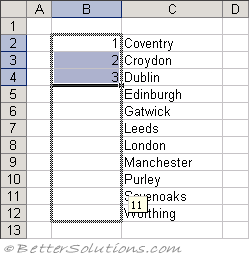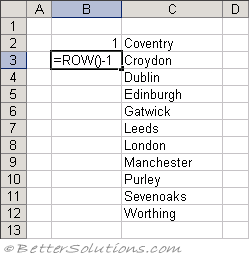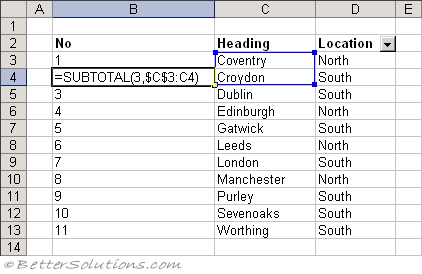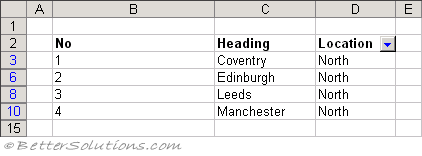# Dynamic Numbering

### Using Static Numbering

This example shows you how to create a dynamic list of numbers that will allow you to delete and insert rows and maintain the correct numbering.
If you want to have numbering down the side of a list to be able to quickly identify the items in the list.
This is the obvious method which involves just entering the numbers into the cell.
To fill a column of cells with increments of a single value, press Ctrl while you drag the fill handle in the bottom right corner.
If the list is quite short you could either just type the numbers in manually or use the Auto Fill feature.
You can use Auto Fill to enter the sequence of numbers.
Just type in the first three numbers (1,2,3) and then use the fill handle in the bottom right corner to drag the cells down.### Using Dynamic Numbering

There will be times when you will want to remove and insert additional rows in which case static numbering is not appropriate.
Instead of using numbers you can use a formula to calculate the correct number.
=ROW() - 1
This formula uses the row number of the current cell to determine the correct number.
The number subtracted from the row number is the number of rows before the list starts.
Therefore if your list started on row 10, then you would subtract 9 from the ROW() number.This method allows you to delete rows and maintain the correct numbering.
You can easily insert rows as well, just remember to copy the formula down from the cell directly above.

### Using Dynamic "Filtered" Numbering

You can use the SUBTOTAL worksheet function to create consecutive numbering of only the visible rows.
The numbering will automatically adjust as you filter or hide various rows.
If your filtered list is in column "A" and your field heading is in row 1, then add the formula to cell A2 and drag it down.
=SUBTOTAL(3, \$C\$3:C3)
Enter the above formula into the cell "B3".Filter the data table to display only the rows that have "North" in column "D".You will notice that the numbers in column "B" are changed automatically.